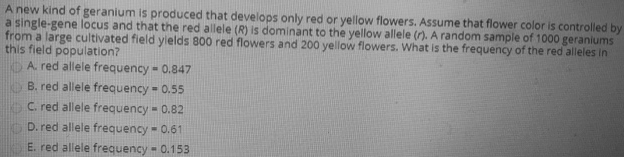# Problem: A new kind of geranium is produced that develops only red or yellow flowers. Assume that flower color is controlled by a single-gene locus and that the red allele (R) is dominant to the yellow allele (r). A random sample of 1000 geraniums from a large cultivated field yields 800 red flowers and 200 yellow flowers. What is the frequency of the red alleles in this field population?A. red allele frequency = 0.847 B. red allele frequency = 0.55C. red allele frequency = 0.82D. red allele frequency = 0.61E. red allele frequency = 0.153

###### FREE Expert Solution

The given problem shows that the phenotypic frequency is 0.8 for the dominant trait and 0.2 for the recessive trait. From the given, we can compute for the allele frequencies only by assuming that the population follows Hardy-Weinberg equilibrium. In a Hardy-Weinberg equilibrium, the frequency of the dominant allele can be represented by p, and the frequency of the recessive allele can be represented by q. For a trait that only has two alleles, p + q = 1. Furthermore, these values can be used to compute for the genotypic frequencies: p2 is for the homozygous dominant, 2pq is for the heterozygotes and q2 for the homozygous recessive.###### Problem Details

A new kind of geranium is produced that develops only red or yellow flowers. Assume that flower color is controlled by a single-gene locus and that the red allele (R) is dominant to the yellow allele (r). A random sample of 1000 geraniums from a large cultivated field yields 800 red flowers and 200 yellow flowers. What is the frequency of the red alleles in this field population?

A. red allele frequency = 0.847

B. red allele frequency = 0.55

C. red allele frequency = 0.82

D. red allele frequency = 0.61

E. red allele frequency = 0.153